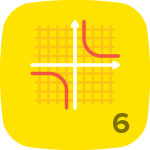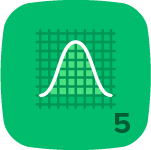# Nghi N.

Retired math tutor. Author of a few math articles, being posted on Google, Bing, Yahoo Search: - The new Transforming Method to solve quadratic equation. - The new non-factoring AC Method to solve quadratic equations - The new Transposing Method to solve linear equations. - The new AC method to factor trinomials. - Solving trig equations, concept and methods. - Solving trig inequalities, concept, methods and steps. - Best method to solve quadratic inequalities